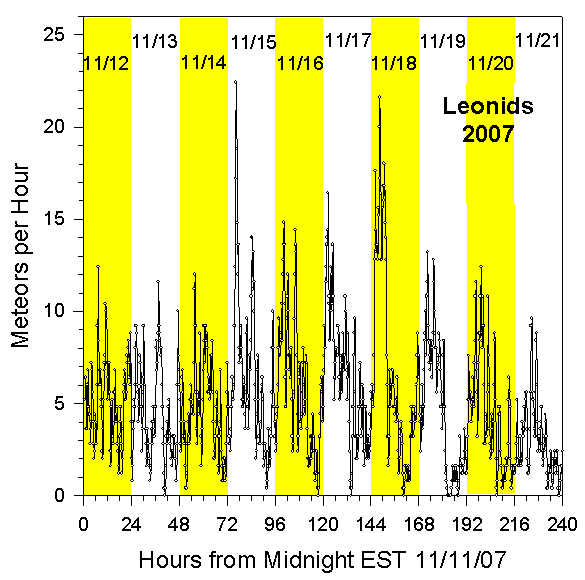Radio Observation of the 2007 Leonids

Data Collection and Analysis

For details about our equipment and method of data analysis, see our main page. There were two peaks. One was at 4:00 AM EST on November 15, 2007, with slightly more than 22 meteors per hour. The other was at 4:00 AM EST on November 18, 2007, with slightly less than 22 meteors per hour. The second peak was at the expected time, but the first peak was unexpected. However, this meteor shower has been quite diffuse in recent years, and there is always considerable activity in the surrounding days.

In the following summary graph, we've counted meteors in quarter hour intervals, and then applied a data smoothing equation of the form

Smoothed_Rate(t) = 3.2*[((1/2)*N(t))+((1/4)*(N(t+1))+((1/4)*(N(t-1))+((1/8)*(N(t+2))+((1/8)*(N(t-2))]

where N(t) is the number of meteors in the fifteen minute interval t, N(t-1)is the number of meteors in the preceding fifteen minute interval, and so on.

Rates for 2007 Leonids

The following graph shows the meteor count rates per hour we measured for the 2007 Leonids. These rates were determined by a computer program that counted all peaks that climbed at least 20% above the background level in one second. The graph shows the rates observed for the ten day interval starting at Midnight EST on 11/11/07.Summary

The 2007 Leonid meteor shower had two peaks. The first was at 4:00 AM EST on November 15, 2007, with slightly more than 22 meteors per hour. The second was at 4:00 AM EST on November 18, 2007, with slightly less than 22 meteors per hour. The second peak was at the expected time, but the first peak was unexpected. However, this meteor shower has been quite diffuse in recent years, and there is always considerable activity in the surrounding days.